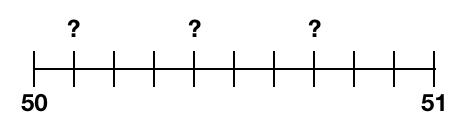# Practice – Basic concepts of decimals

Questions :

Study the number line given below. There are a few unknown numbers represented by question marks. What number does each question mark represent? Write your answer both in fractions and decimals.Study the number line given below. There are a few unknown numbers represented by question marks. What number does each question mark represent? Write your answer both in fractions and decimals.Study the number line given below. There are a few unknown numbers represented by question marks. What number does each question mark represent? Write your answer both in fractions and decimals.Study the number line given below. There are a few unknown numbers represented by question marks. What number does each question mark represent? Write your answer both in fractions and decimals.Study the number line given below. There are a few unknown numbers represented by question marks. What number does each question mark represent? Write your answer both in fractions and decimals.Study the following set of numbers. Draw a number line on your notebook and place these numbers on the number line. Also, denote the numbers as fractions on the number line.

0.8, 0.6 and 0.4

Study the following set of numbers. Draw a number line on your notebook and place these numbers on the number line. Also, denote the numbers as fractions on the number line.

#### 2.2, 2.4 and 2.9

Study the following set of numbers. Draw a number line on your notebook and place these numbers on the number line. Also, denote the numbers as fractions on the number line.

#### 10.5, 10.6 and 10.8

Study the statement below. Study the position of zero and say if the statement is correct or false.

(REMEMBER: If a decimal number ends with zero then that zero does not have any meaning)

0.1 = 0.10 (True or false?)

0.2 = 0.200 (True or false?)

0.31 = 0.301 (True or false?)

0.2 = 0.02 (True or false?)

0.03 = 0.003 (True or false?)

2 = 02 (True or false?)

2 = 20 (True or false?)

Explanations :Video Explanation :0.1 = 0.10   TRUE (the right most 0 in 0.10 has no meaning)

0.2 = 0.200  TRUE

0.31 = 0.301  FALSE ( The 0 in the middle has meaning. zero does not have any meaning in decimals only if the number is ending with zeros)

0.2 = 0.02 FALSE

0.03 = 0.003 FALSE

2 = 02 TRUE ( For whole number, zero in the left of a number has no meaning)

2 = 20 False ( Obviously !!)

Scroll to Top